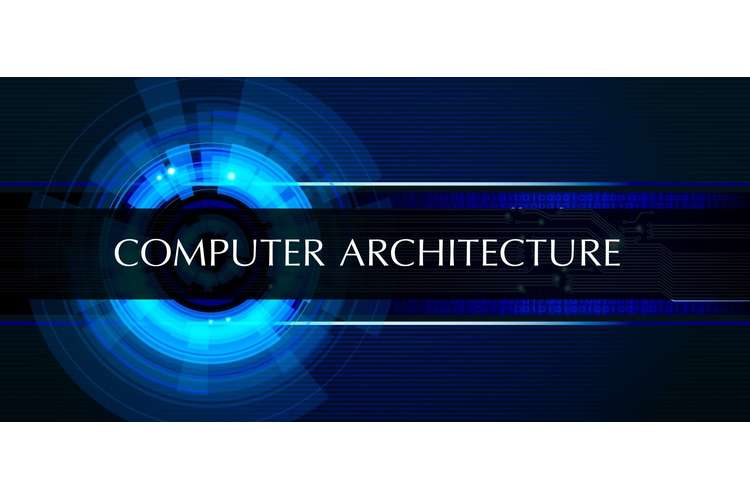ITC544 Computer Organization and Architecture Editing ServicesITC544 Computer Organization and Architecture Editing Services

This assessment gives the knowledge of the function and designs many computer system components essential to procedure information digitally. It improves understanding of computer hardware and computer organization.

Learning outcomes

After submission of this assessment, you should be able to:

1. Show and use terminologies of computer organization and architecture

2. implement an understanding of data representations and calculations to practical situations;

3. implement digital logic and Boolean algebra and to plan & construe multifaceted digital circuits;

4. examine the internal operation of the CPU and define how it is used to complete instructions;

Peer Wise

The value of this assessment is 10%. For this assessment item, you need to register in the PeerWise system.

If you have used PeerWise before, simply log in and then select "Join course" from the Home menu. To access the Part I of the Assessment Item 1, "ITC544 Assessment Item 1 Part I", you will need to enter two pieces of information: (5 marks)

1. Course ID = To be provided on the Interact2 site – Announcement

2. Identifier = Please enter your CSU Student ID for this course

To access the Part II of the Assessment Item 1, "ITC544 Assessment Item 1 Part II", you will need to enter two pieces of information (5marks)

1. Course ID = To be provided on the Interact2 site – Announcement

2. Identifier = Please enter your CSU Student ID for this course

Plagiarism Quiz

In this task, students need to take on and complete the online quiz that covers questions regarding plagiarism and referencing. Students may attain the passing score of 18 from 20.

Data Representation & Digital Logic

The value of this assessment is 30 marks.

Q.1 a. Determine the value of base x if (211)x = (6A)16 [5 marks]

b. Convert the followings: [3+3=6 marks]

58810 into a 3-base number

c. Given a (very) tiny computer that has a word size of 6 bits, what are the smallest negative numbers and the largest positive numbers that this computer can represent in each of the following representations? [3 +3 = 6 marks]

One's complement
Two's complement

Q.2 a. Consider the following logic diagram of a combinational circuit where A, B, and C are inputs and Q is the output. Three 2-input AND gates and two 2-input OR gates are used in the circuit. It is possible to reduce some of the logic gates without changing the functionality of the circuit. Such component reduction results in higher operating speed (less delay time from input signal transition to output signal transition), less power consumption, less cost, and greater reliability. Construct a logic diagram of a circuit which does have the same function output with only two logic gates (instead of five). Please show the steps. [8 marks]

b. Using basic Boolean algebra identities for Boolean variables A, B and C, prove that ABC+ ABC' + AB'C + A'BC = AB + AC + BC. Please show all steps and mention the identities used. [5 marks]

MARIE and ISA

The value of this assignment is 15%.

1. a. The Fibonacci numbers are the numbers in the following integer sequence, called the Fibonacci sequence, and are characterized by the fact that every number after the first two is the sum of the two preceding ones: 0, 1, 1, 2, 3, 5, 8, 13, 21, 34, 55, 89, 114, … etc.

By definition, the first two numbers in the Fibonacci sequence are 0 and 1, and each subsequent number is the sum of the previous two. We define Fib(0)=0, Fib(1)=1, Fib(2)=1, Fib(3)=2

Fib(4)=3, etc. The first 22 Fibonacci numbers given below:

 Fib(0) Fib(2) Fib(2) Fib(3) Fib(4) Fib(5) Fib(6) Fib(7) Fib(8) Fib(9) Fib(10) 0 1 1 2 3 5 8 13 21 34 55
 Fib(11) Fib(12) Fib(13) Fib(14) Fib(15) Fib(16) Fib(17) Fib(18) Fib(19) Fib(20) Fib(21) 89 144 233 377 610 987 1597 2584 4181 6765 10946

Write a MARIE program to calculate Fib (n), where the user inputs n. For example, if the user inputs 7, the program output the value 13; if the user inputs 15, the program outputs the value 610; if the user inputs 20, the program outputs the value 6765 etc. You need to write and run the program using MARIE simulator. Please include appropriate comments to make your code readable.[10 marks]

b. For some values of n, your program will not produce correct results. You can check this by gradually increasing the values of n and checking for the correct outputs. What is the maximum value of n for which your program produces a correct result? Why? Please comment on this [5 marks].

2. You are designing an instruction set for your computer. All the instructions are of same size (11 bits long). The size of an address field is 4 bits. You have already designed 5 2-address instructions and 45 1-address instructions. How many 0-address instructions still you can fit? Justify your answer. [7 marks]

3. Write codes to implement the expression: A= (B + C * ) on 3-, 2-, 1- and 0-address machines. In accordance with programming language practice, computing the expression should not change the values of its operands. [8 marks]